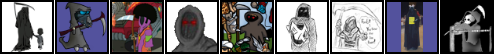# Chemistry Boyle's Law and Log10 of Volume and Pressure

Alright, so taking a second semester of general chemistry. We're getting deeper into gas laws, which is all pretty straightforward. Part of our lab this past week was to verify Boyle's Law which included measuring volume vs pressure using Microlab, recording and graphing the data and also creating a graph of the Log base 10 of both volume and pressure. I'm not clear on what that Log10 relationship is showing me. What was I supposed to infer from that data or use as validation of Boyle's Law? My google-fu is failing me on this one.

## Posts

• Death Lite, Only 1 Calorie Registered User, ClubPA regular
I'm not a chemist so this is a guess, but it might help point you in the right direction.

I'm guessing the overall point of the exercise was both to demonstrate Boyle's Law, but also to teach you how to look for such relationships.

Basically trying to get you to mentally create the animated gif on the Wikipedia page. Presumably to get you to extrapolate something like this demonstration of the relationship

I'm pretty sure (but might be wrong because it's been a long time) that using a log scale on both axis of the graph won't change the relationship, just allow you to see the trend extending across a much wider range of values.

So my guess is that the log is not critical to the relationship you're supposed to be seeing, but rather just a way to allow you to see it across values at different orders of magnitude. So to help you see it's not just a small scale trend but universal.• Boyle's law is pretty much PV = nRT. Boyle's law just uses PV = k, provided there is a fixed value of temperature. If I remember right, moles didn't exist as a concept at that point.

You can also write that as P = k/V. Then you can take the log10 of both sides. I show natural log below.

ln (P) = ln (k/V)

ln (P) = ln (k) - ln (V)

So a plot of ln (P) vs. ln(V) should have a slope of -1 with an intercept at ln(k).

The transformation is usually done to minimize fitting error.

3DS Friends: 1693-1781-7023
• Ah, thanks guys. So the log-log graph is basically just showing that as volume increases, the actual value of pressure change is Z% of the previous pressure change and we've got a line with each plot point getting slightly closer together and the distance between shrinking by the same relative amount each time, so each section between plots points is 80% of the length of the previous one or whatever.

Now to figure out a good way to word this for the lab report, but I think I'm all set now... until I have to ask about the next thing.